# Erosion and Dilation in Digital Image Processing

## Morphological Image Processing:

It deals with extracting image components that are useful in representation and description of shape. It includes basic morphological operations like erosion and dilation. The outputs of morphological processing generally are image attributes.

Erosion and dilation are fundamental morphological operations. But before understanding these, let us see some basics which are essential to understanding erosion and dilation:

### Translation:

• The translation of set ‘A’ by points z = (z1,z2) is given by: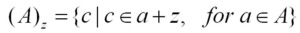It simply means that (x,y) —–> (x+z, y+z2)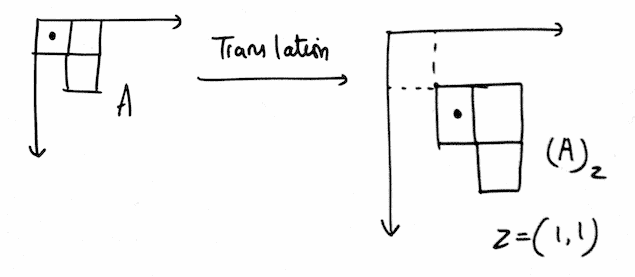As seen from the above figure, we can see a point A(x,y) is translated by z(1,1)

### Reflection:

• The reflection of set ‘B’ about the origin of B is given by: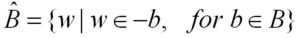It simply means that (x,y) —–> (-x , -y)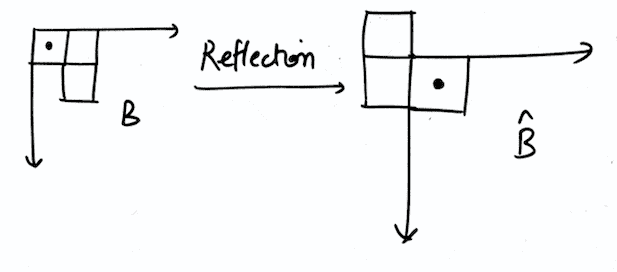### Structuring Element (SE):

• Structuring elements are small sets or sub-images used to probe (examine) an image under study for properties of interest.
• These can be of any size and make any shape.
• For simplicity, we use rectangular structuring element with their origin at the middle pixel. Origin of SE is marked by a dot (•).
• Structuring elements do not require numerical values.
• SE is moved across every pixel in the original image to give a pixel in a new processed image.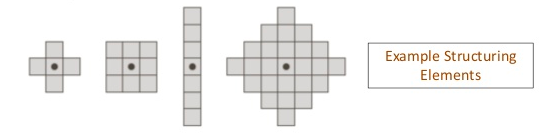### Erosion in Morphological Image Processing:

For sets A and B in Z2 (Binary Image), erosion of A by B is denoted by A⊖B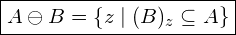It is the set of all points z such that B, shifted or translated by z, is contained in A.

• It is used for removing irrelevant size details from a binary image.
• Erosion shrinks the image.
• It can strip away extrusions.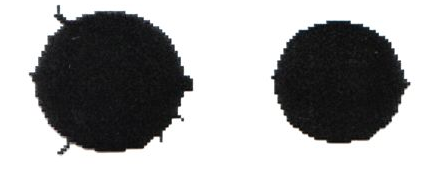• It can strip apart joined objects.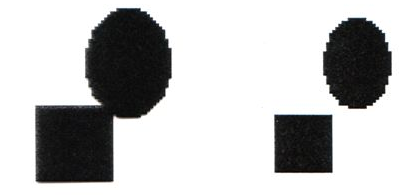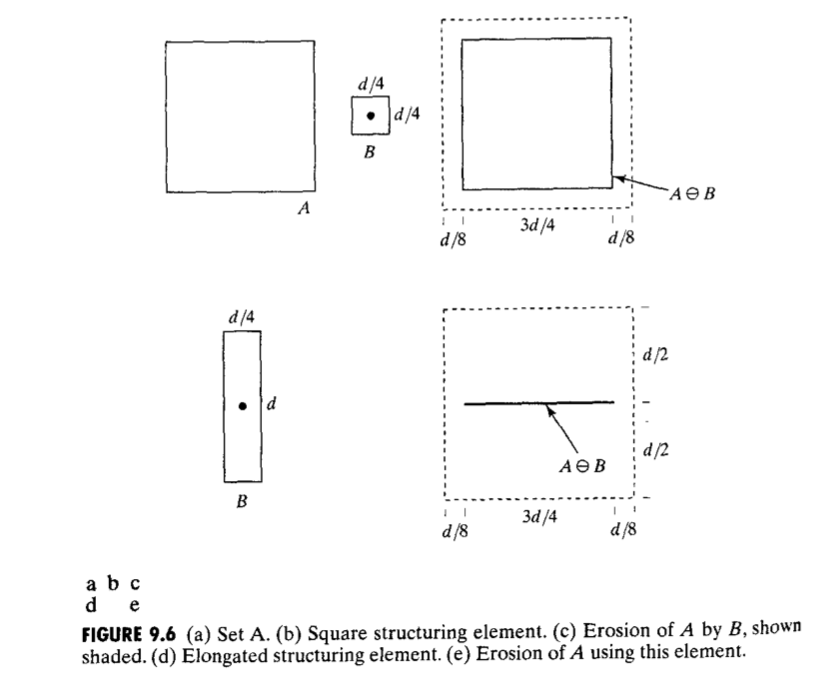### Dilation in Morphological Image Processing:

For sets A and B in Z2 (Binary Image), dilation of A by B is denoted by A⊕B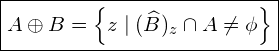In dilation, first B is reflected about its origin by 180°, then this reflection is translated by z, then A⊕B is a set of all displacement z such that it has at least one of its pixels contained in A.

• It enlarges the image.
• It is used for bridging gaps of character due to poor resolution.
• Dilation can repair breaks.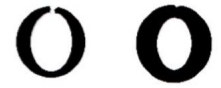• It can repair intrusions.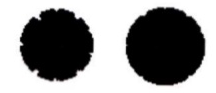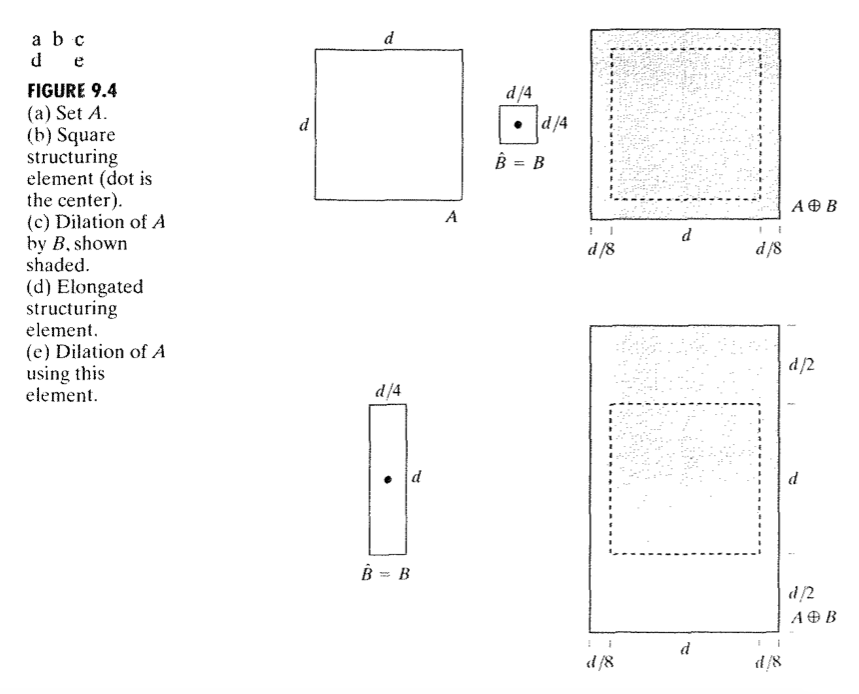Some Important Points:
• Erosion and dilation are dual with respect to set complementation and reflection to each other.
• Dilation expands an image and erosion shrinks the image.

References:

This site uses Akismet to reduce spam. Learn how your comment data is processed.

error: